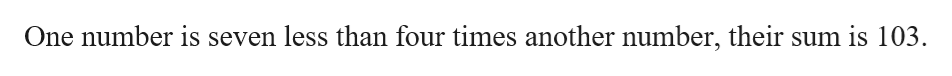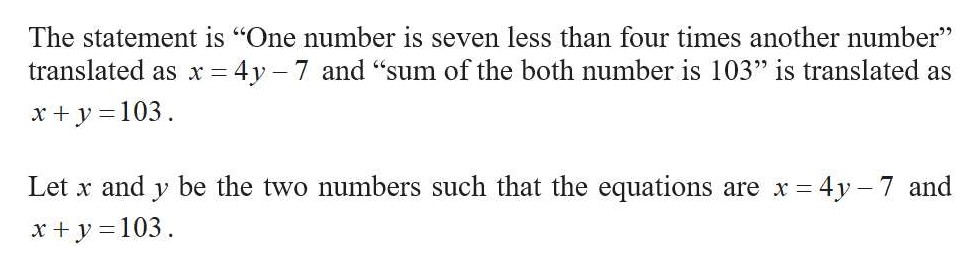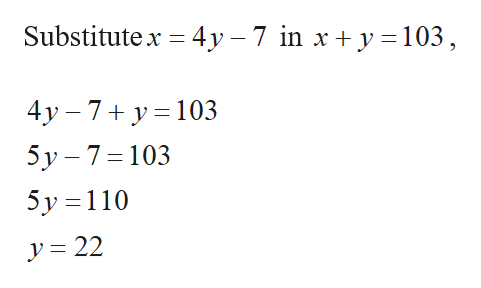# One number is seven less than four times another number. If their sum is 103. Find both numbers.

Question
1 views

One number is seven less than four times another number. If their sum is 103. Find both numbers.

check_circle

Step 1

The given statement ishelp_outlineImage TranscriptioncloseOne number is seven less than four times another number, their sum is 103. fullscreen
Step 2

Translate the statement as equation.help_outlineImage TranscriptioncloseThe statement is "One number is seven less than four times another number" - 4y 7 and "sum of the both number is 103" is translated as translated as x y 103 Let x and y be the two numbers such that the equations 4y 7 and are x = xy 103 fullscreen
Step 3

Find the values of &nb...help_outlineImage TranscriptioncloseSubstitutex 4y -7 in x + y 103, 4y 7 y 103 5y 7 103 5y 110 y=22 fullscreen

### Want to see the full answer?

See Solution

#### Want to see this answer and more?

Solutions are written by subject experts who are available 24/7. Questions are typically answered within 1 hour.*

See Solution
*Response times may vary by subject and question.
Tagged in

### Other